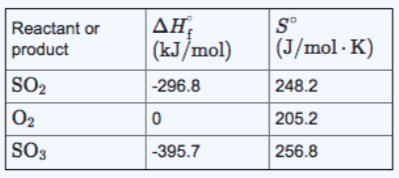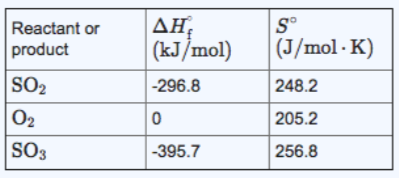# Problem: Consider the oxidation of SO2 to SO3: SO2 (g) + 1/2 O2 (g) → SO3 (g) Calculate ΔGºrxn at 25 C. Express your answer to one decimal place with the appropriate units. Determine whether the reaction is spontaneous at standard conditions.

###### FREE Expert Solution
86% (484 ratings)
###### FREE Expert Solution

The standard free energy change of a reaction (ΔG˚rxn) is given by the following equation:The balanced equation for the oxidation of SO2 is:

SO2(g) + 1/2 O2(g)  SO3(g)

We’re given the ΔH˚f and S˚ of each reactant and product. We can use those to calculate for ΔH˚rxn and ΔS˚rxn separately.86% (484 ratings)###### Problem Details

Consider the oxidation of SO2 to SO3

SO2 (g) + 1/2 O2 (g) → SO3 (g)

Calculate ΔGºrxn at 25 C.Determine whether the reaction is spontaneous at standard conditions.

What scientific concept do you need to know in order to solve this problem?

Our tutors have indicated that to solve this problem you will need to apply the Gibbs Free Energy concept. You can view video lessons to learn Gibbs Free Energy. Or if you need more Gibbs Free Energy practice, you can also practice Gibbs Free Energy practice problems.

What is the difficulty of this problem?

Our tutors rated the difficulty ofConsider the oxidation of SO2 to SO3: SO2 (g) + 1/2 O2 (g) →...as medium difficulty.

How long does this problem take to solve?

Our expert Chemistry tutor, Dasha took 8 minutes and 49 seconds to solve this problem. You can follow their steps in the video explanation above.

What professor is this problem relevant for?

Based on our data, we think this problem is relevant for Professor Perrotta's class at UCM.